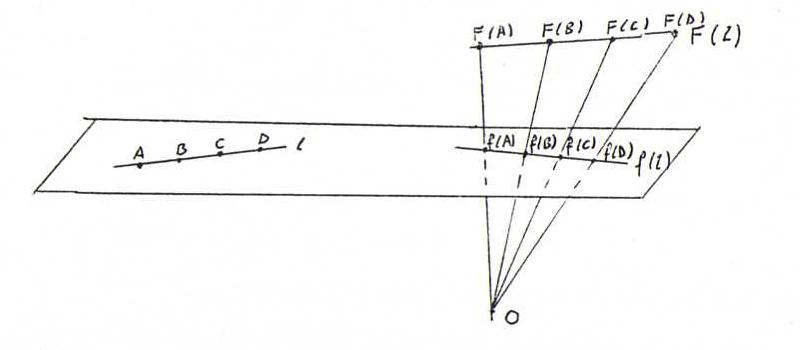### COURSE OF PROJECTIVE GEOMETRY

O39 Dual fundamental theorem; given three lines a, b, c through a point L and three lines a', b', c' through a point M (possibly L = M), there exists axactly one projectivity ψ from the pencil of lines L onto the pencil of lines M with ψ(a) = a', ψ(b) = b' and ψ(c) = c'.
Proof: Let p be a straight line, not through L or M. Denote X:=x.p for all x through L, and X ' :=x'.p for all x' throughr M.
Let φ be the projectivity from p onto p with φ(A) = A', φ(B) = B', φ(C) = C'.
Let δ be the elementary perspectivity from L onto p, and ε the elementary perspectivity from M onto p. Then ψ := ε-1φδ meets our requirements.
Suppose that ψ' does the same. Then φ' := εψ'δ-1 is a projectivity from p onto p with φ'(A) = A', φ'(B) = B', φ'(C) = C'. So φ = φ'. But then ψ' = ε-1φδ = ψ.

O40 Choose two points A and B on l, both unequal to S. Denote A' := φ(A), B' := φ(B), and let T be the intersection point of AA' and BB'.
Let ψ be the perspectivity from l onto m with center T.
Then ψ(A)=φ(A), ψ(B)=φ(B), ψ(C)=φ(C). According to FT we find ψ = φ.

O41 If c = a + λ(b - a) then F(c) = F(a) + λ(F(b) - F(a)), and || (F(c) - F(a))|| / ||(F(b) - F(a)) || = |λ| = || (c - a) || / || (b - a) ||.
From the picture below we deduce that F preserves cross ratio:(A, B; C, D) = (F(A), F(B); F(C), F(D)) = (f(A), f(B); f(C), f(D)).
The first equality follows from the first part of this answer, the second follows by projection from O.

O42
c = -a + 2b, so λ= -1, μ=2; c' = (1/2)a' + (1/2)b', so ρ = σ = 1/2.
Let x = (0,ξ,1), then x = (2-ξ)a + (ξ-1)b.
So F(x) = (1/2)(ξ-2)a' + (1/4)(ξ-1)b' = ((3-ξ)/4, 0, (3ξ-5)/4). So f(x) = ((3-ξ)/(3ξ-5), 0, 1).
We find (A, B; C, X) = (2ξ-4)/(ξ-1) and (A', B'; C', X') = (1-ξ')/(1+ξ'). With ξ' = (3-ξ)/(3ξ-5) it follows that (A, B; C, X) = (A', B'; C', X').